# Propositional Logic Propositional Languages A propositional signature is

• Slides: 32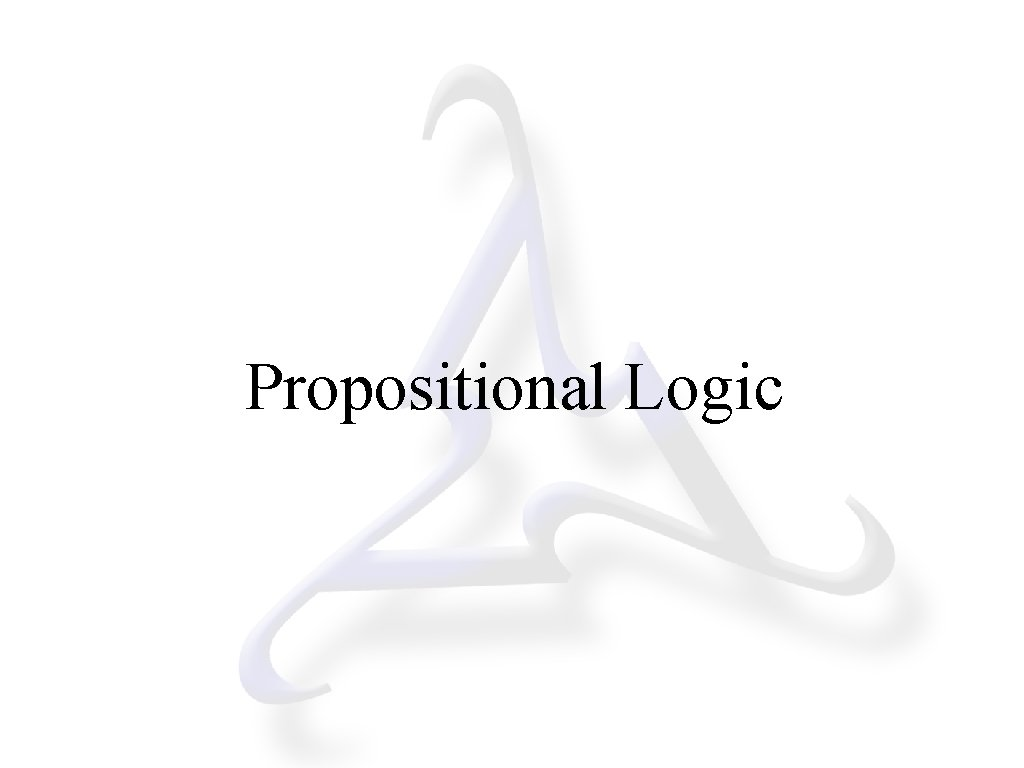Propositional LogicPropositional Languages A propositional signature is a set/sequence of primitive symbols, called proposition constants. Given a propositional signature, a propositional sentence is either (1) a member of the signature or (2) a compound expression formed from members of the signature. (Details to follow. ) A propositional language is the set of all propositional sentences that can be formed from a propositional signature. 2Proposition Constants By convention (in this course), proposition constants are written as strings of alphanumeric characters beginning with a lower case letter. Examples: raining r 32 aining r. Ai. Ng rainingorsnowing Non-Examples: 324567 raining. or. snowing 3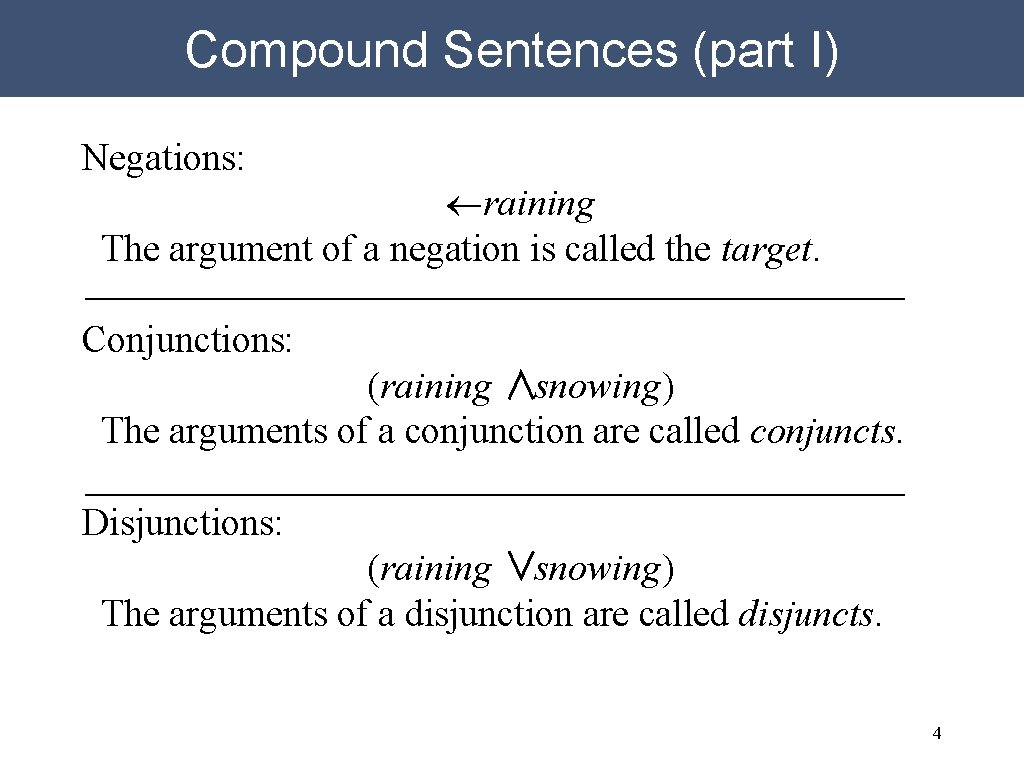Compound Sentences (part I) Negations: ¬raining The argument of a negation is called the target. Conjunctions: (raining ∧snowing) The arguments of a conjunction are called conjuncts. Disjunctions: (raining ∨snowing) The arguments of a disjunction are called disjuncts. 4Compound Sentences (part II) Implications: (raining ⇒cloudy) The left argument of an implication is the antecedent. The right argument is the consequent. Equivalences: (cloudy ⇔raining) 5Parentheses Removal Dropping Parentheses is good: (p ∧q) →p ∧q But it can lead to ambiguities: ((p ∨q) ∧r) →p ∨q ∧r (p ∨(q ∧r)) →p ∨q ∧r 6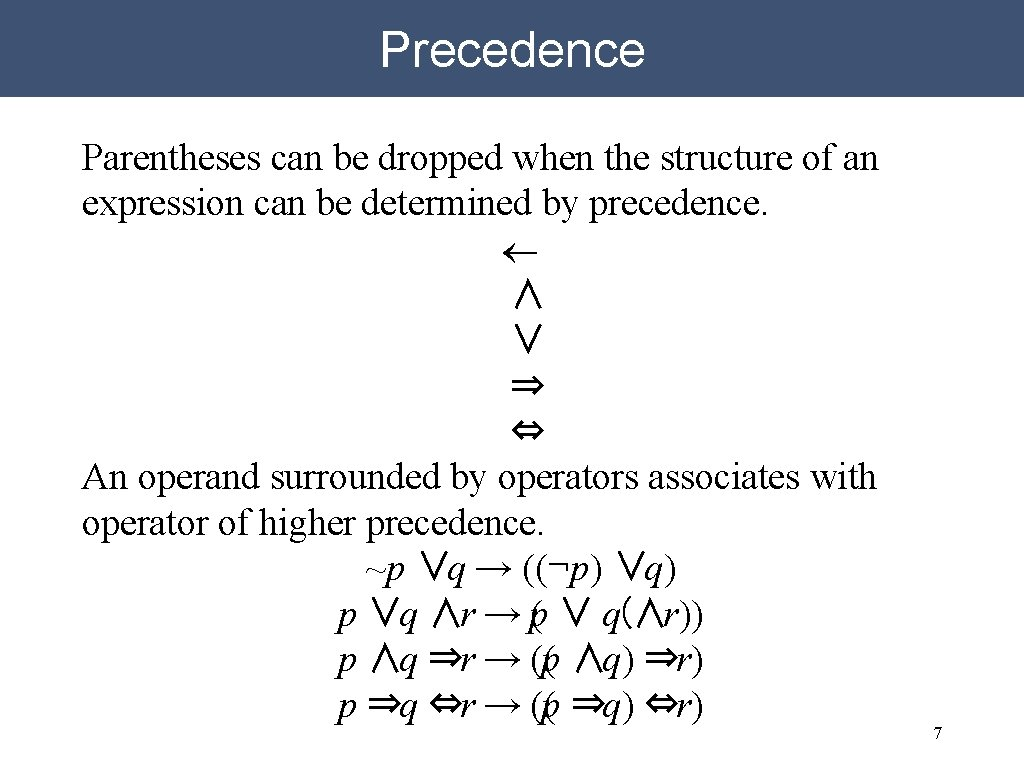Precedence Parentheses can be dropped when the structure of an expression can be determined by precedence. ¬ ∧ ∨ ⇒ ⇔ An operand surrounded by operators associates with operator of higher precedence. ~p ∨q → ((¬p) ∨q) p ∨q ∧r → p( ∨ q(∧r)) p ∧q ⇒r → ((p ∧q) ⇒r) p ⇒q ⇔r → ((p ⇒q) ⇔r) 7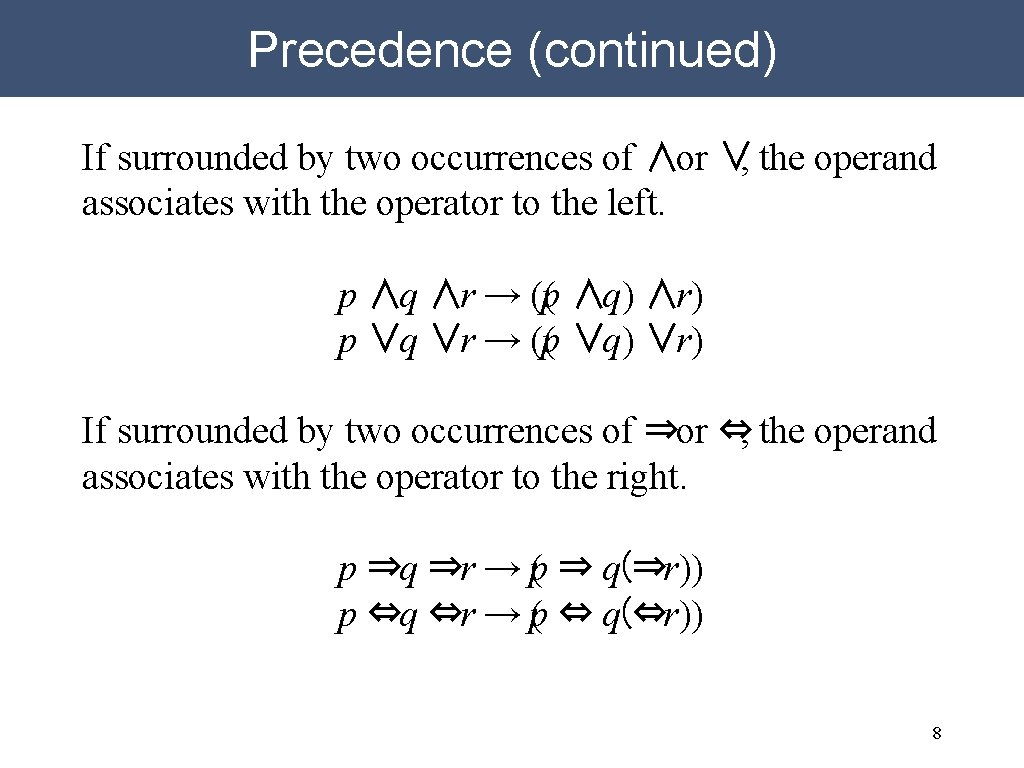Precedence (continued) If surrounded by two occurrences of ∧or ∨, the operand associates with the operator to the left. p ∧q ∧r → ((p ∧q) ∧r) p ∨q ∨r → ((p ∨q) ∨r) If surrounded by two occurrences of ⇒or ⇔, the operand associates with the operator to the right. p ⇒q ⇒r → p( ⇒ q(⇒r)) p ⇔q ⇔r → p( ⇔ q(⇔r)) 8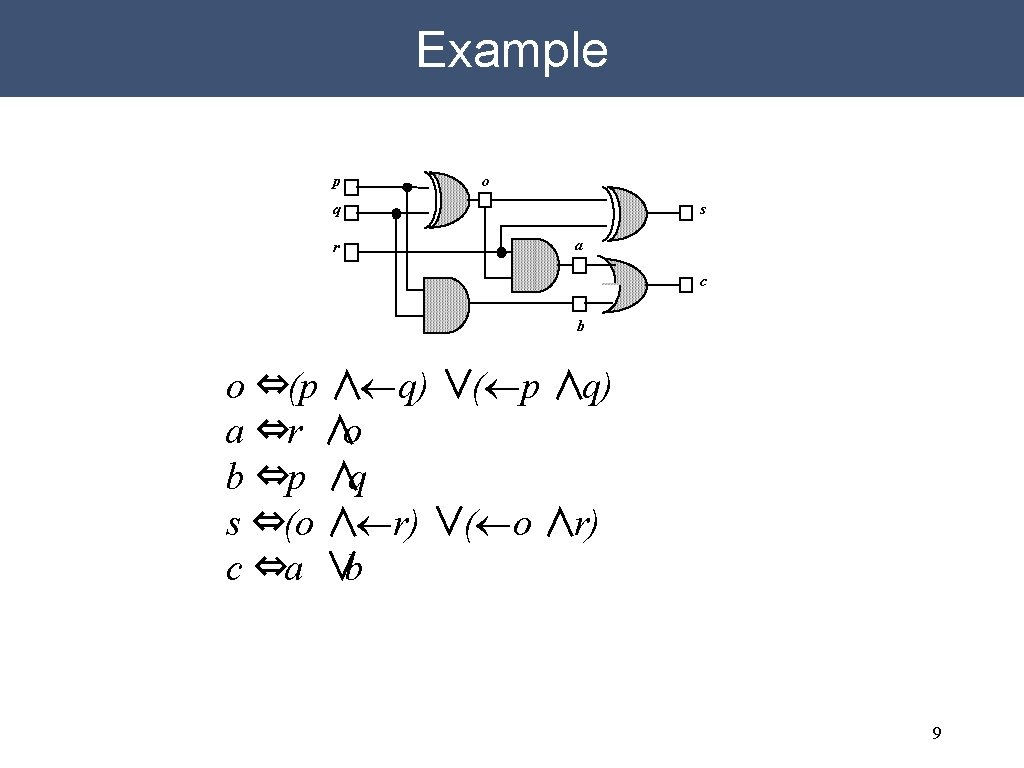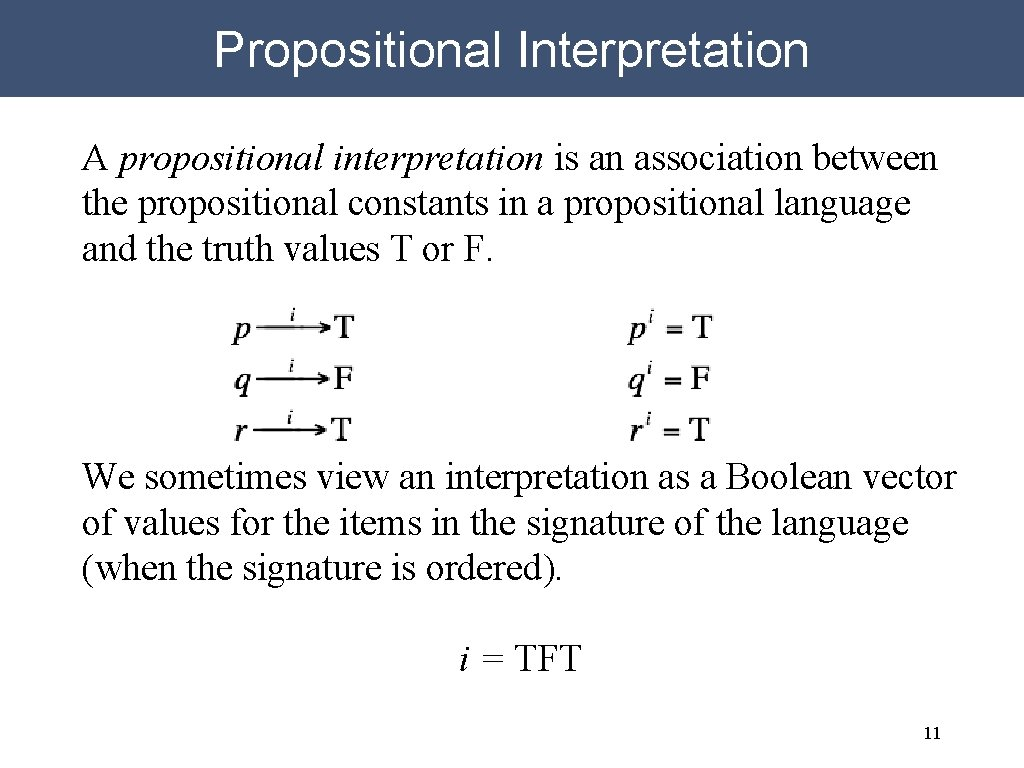Propositional Interpretation A propositional interpretation is an association between the propositional constants in a propositional language and the truth values T or F. We sometimes view an interpretation as a Boolean vector of values for the items in the signature of the language (when the signature is ordered). i = TFT 11Sentential Interpretation A sentential interpretation is an association between the sentences in a propositional language and the truth values T or F. pi = T qi = F ri = T (p ∨q)i = T (¬q ∨r)i = T ((p ∨q) ∧(¬q ∨r))i = T A propositional interpretation defines a sentential interpretation by application of operator semantics. 12Operator Semantics Negation: For example, if the interpretation of p is F, then the interpretation of ¬p is T. For example, if the interpretation of (p∧q) is T, then the interpretation of ¬(p∧q) is F. 13Operator Semantics (continued) Conjunction: Disjunction: NB: The type of disjunction here is called inclusive or, which says that a disjunction is true if and only if at least one of its disjuncts is true. This contrasts with exclusive or, which says that a disjunction is true if and only if an odd number of its disjuncts is true. 14Operator Semantics (continued) Implication: NB: The semantics of implication here is called material implication. Any implication is true if the antecedent is false, whether or not there is a connection to the consequent. If Shakespeare is alive, then 2+2=5. 15Operator Semantics (concluded) Equivalence: 16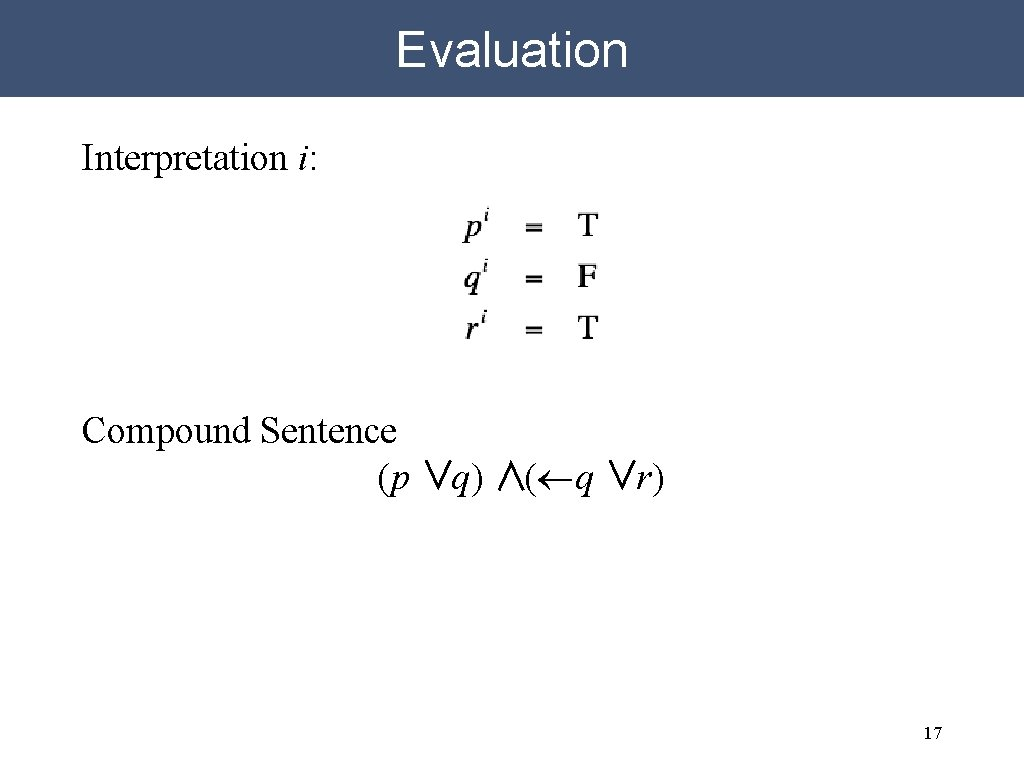Evaluation Interpretation i: Compound Sentence (p ∨q) ∧(¬q ∨r) 17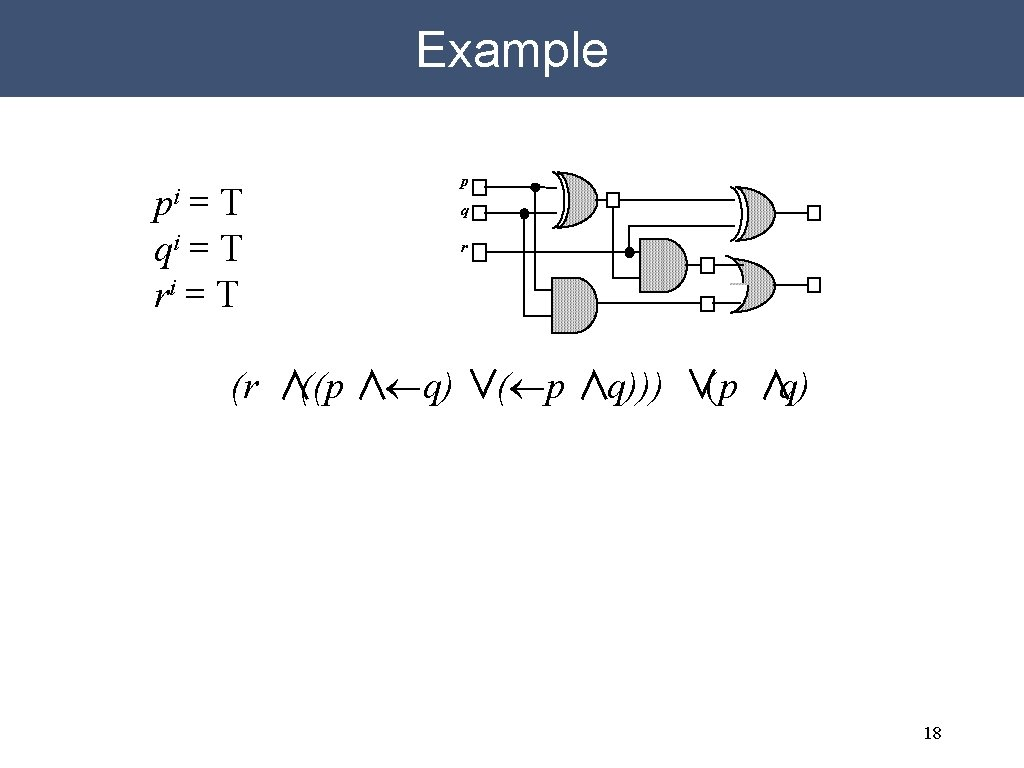Example pi = T qi = T ri = T p q r (r ∧((p ∧¬q) ∨(¬p ∧q))) ∨(p ∧q) 18Multiple Interpretations Logic does not prescribe which interpretation is “correct”. In the absence of additional information, one interpretation is as good as another. Interpretation i Interpretation j Examples: Different days of the week Different locations Beliefs of different people 19Truth Tables A truth table is a table of all possible interpretations for the propositional constants in a language. One column per constant. One row per interpretation. For a language with n constants, there are 2 n interpretations. 20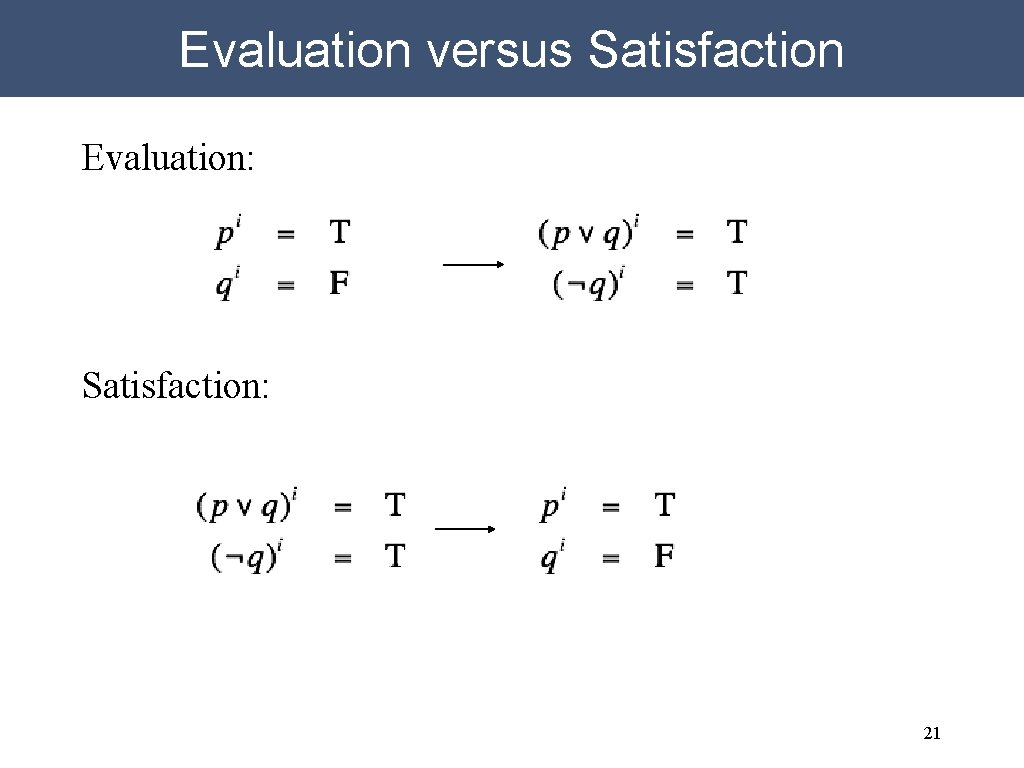Evaluation versus Satisfaction Evaluation: Satisfaction: 21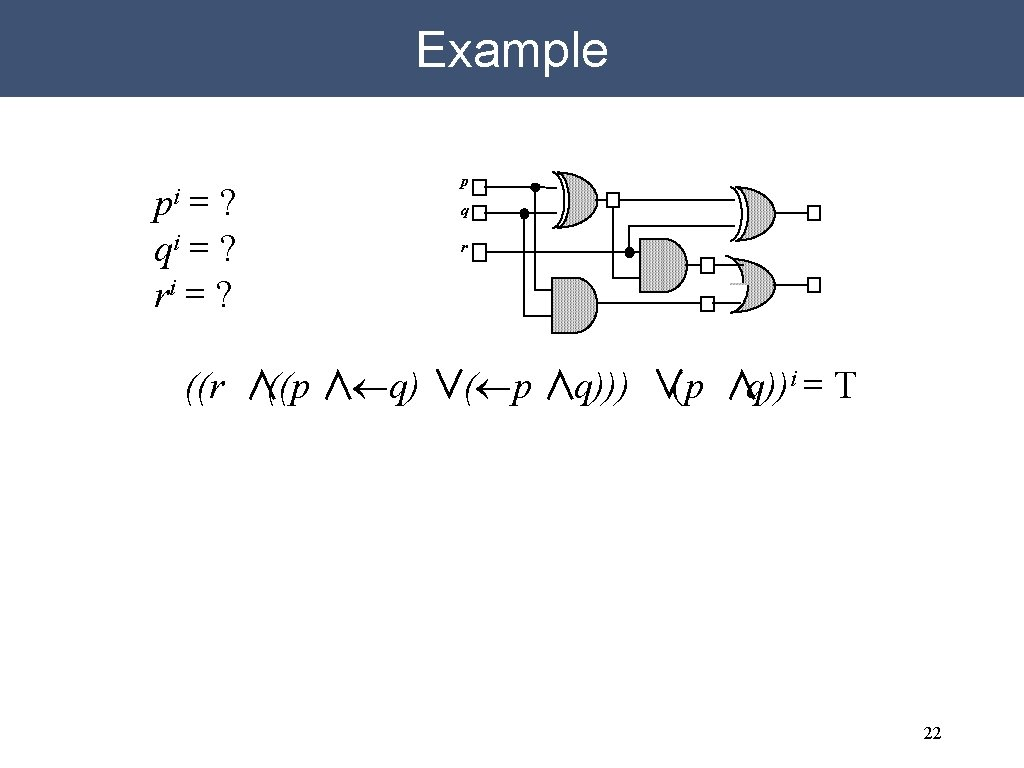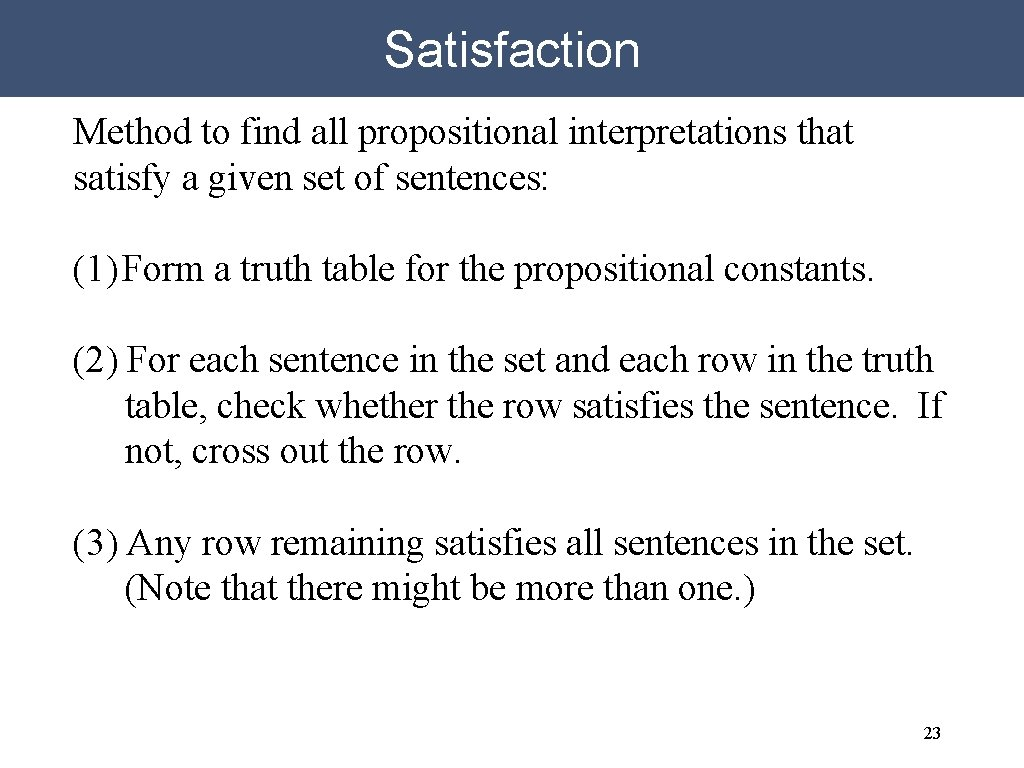Satisfaction Method to find all propositional interpretations that satisfy a given set of sentences: (1) Form a truth table for the propositional constants. (2) For each sentence in the set and each row in the truth table, check whether the row satisfies the sentence. If not, cross out the row. (3) Any row remaining satisfies all sentences in the set. (Note that there might be more than one. ) 23Satisfaction Example q⇒r 24Satisfaction Example (continued) q⇒r p ⇒q∧r 25Satisfaction Example (concluded) q⇒r p ⇒q∧r ¬r 26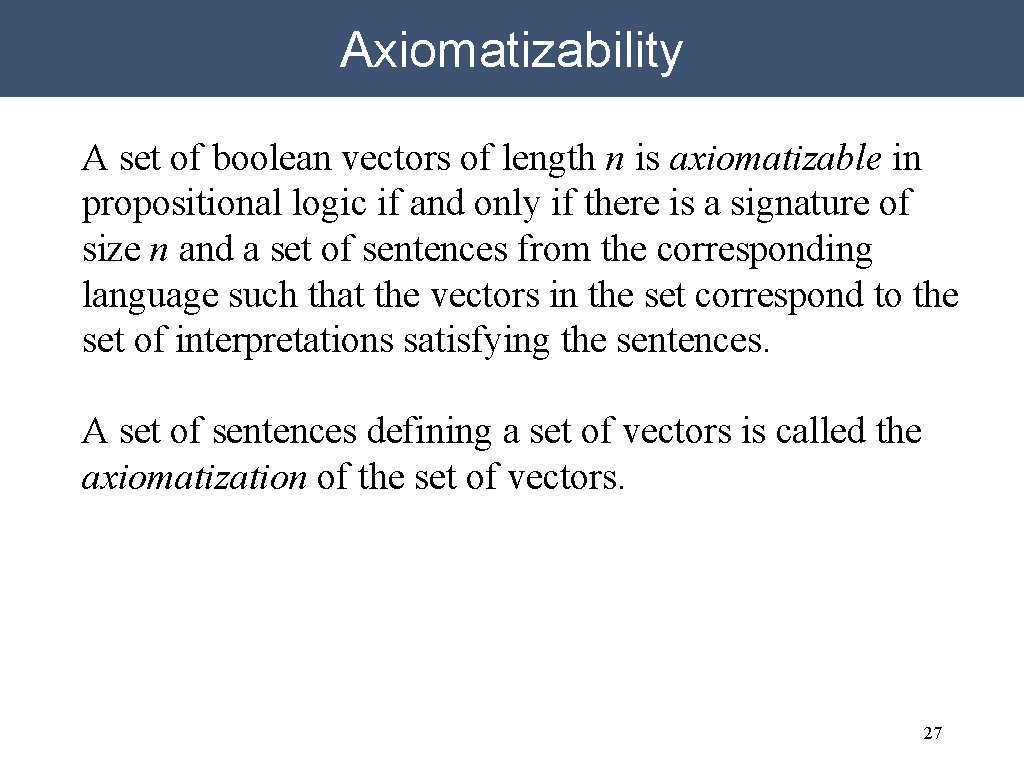Axiomatizability A set of boolean vectors of length n is axiomatizable in propositional logic if and only if there is a signature of size n and a set of sentences from the corresponding language such that the vectors in the set correspond to the set of interpretations satisfying the sentences. A set of sentences defining a set of vectors is called the axiomatization of the set of vectors. 27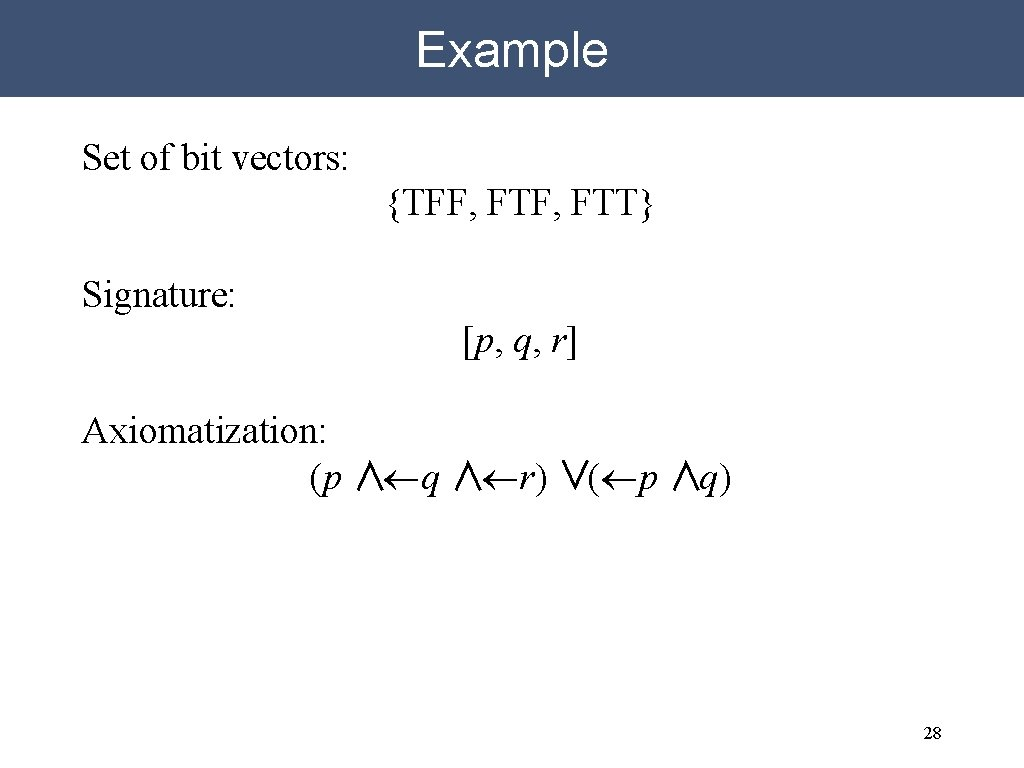Example Set of bit vectors: {TFF, FTT} Signature: [p, q, r] Axiomatization: (p ∧¬q ∧¬r) ∨(¬p ∧q) 28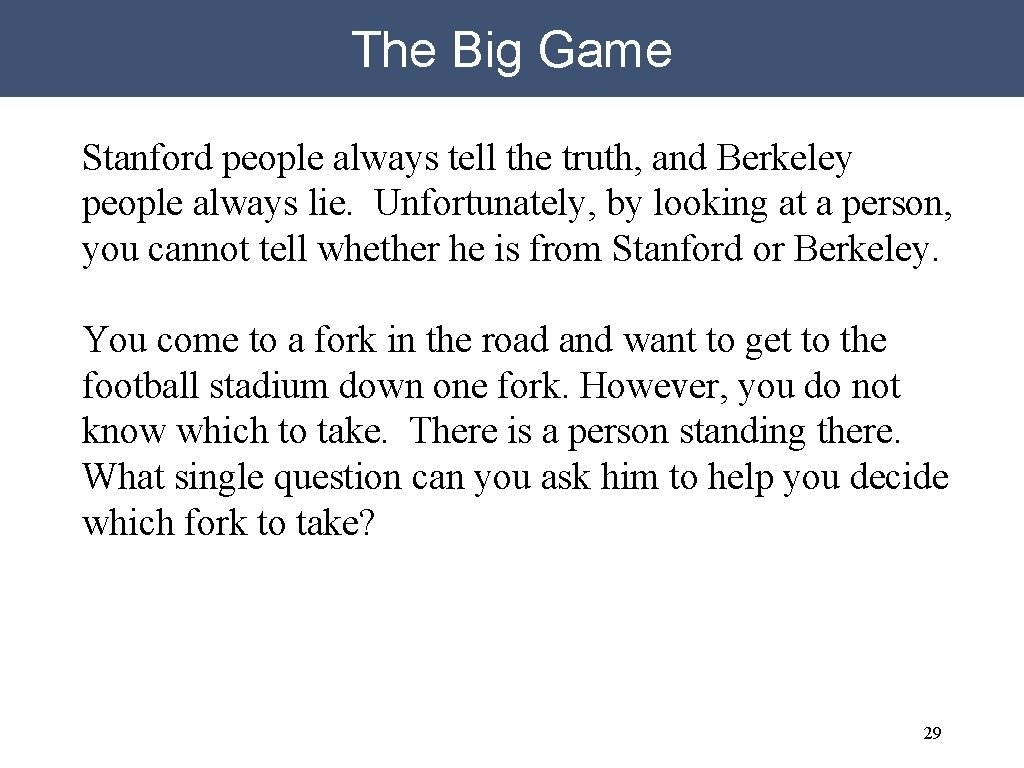The Big Game Stanford people always tell the truth, and Berkeley people always lie. Unfortunately, by looking at a person, you cannot tell whether he is from Stanford or Berkeley. You come to a fork in the road and want to get to the football stadium down one fork. However, you do not know which to take. There is a person standing there. What single question can you ask him to help you decide which fork to take? 29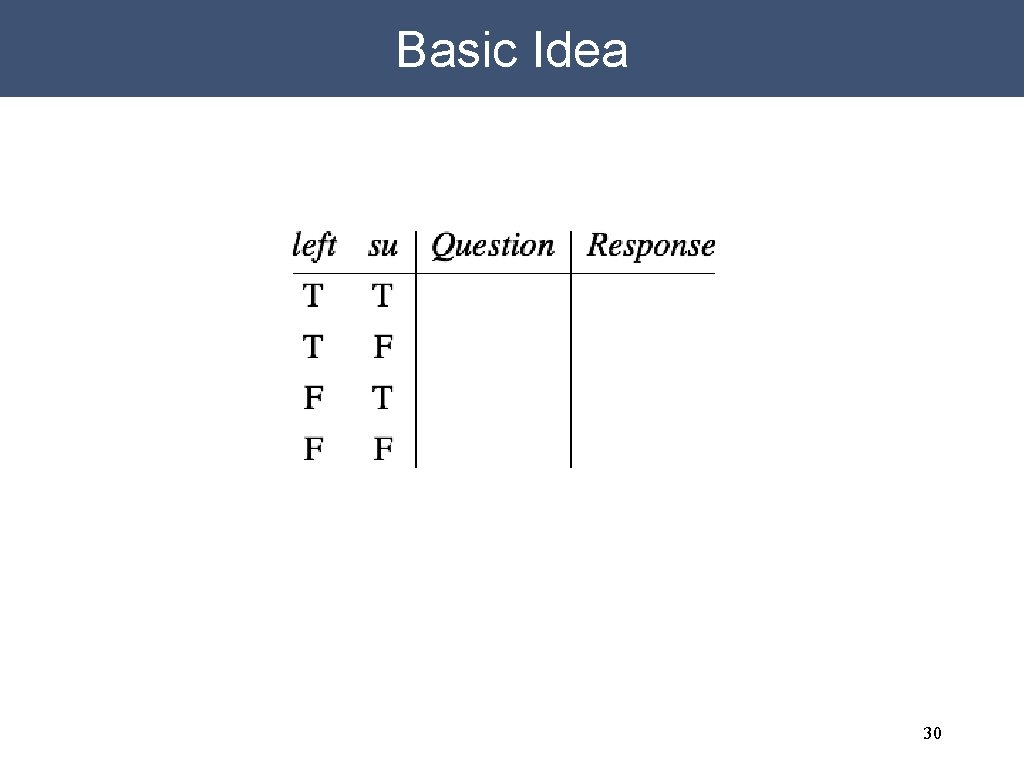Basic Idea 30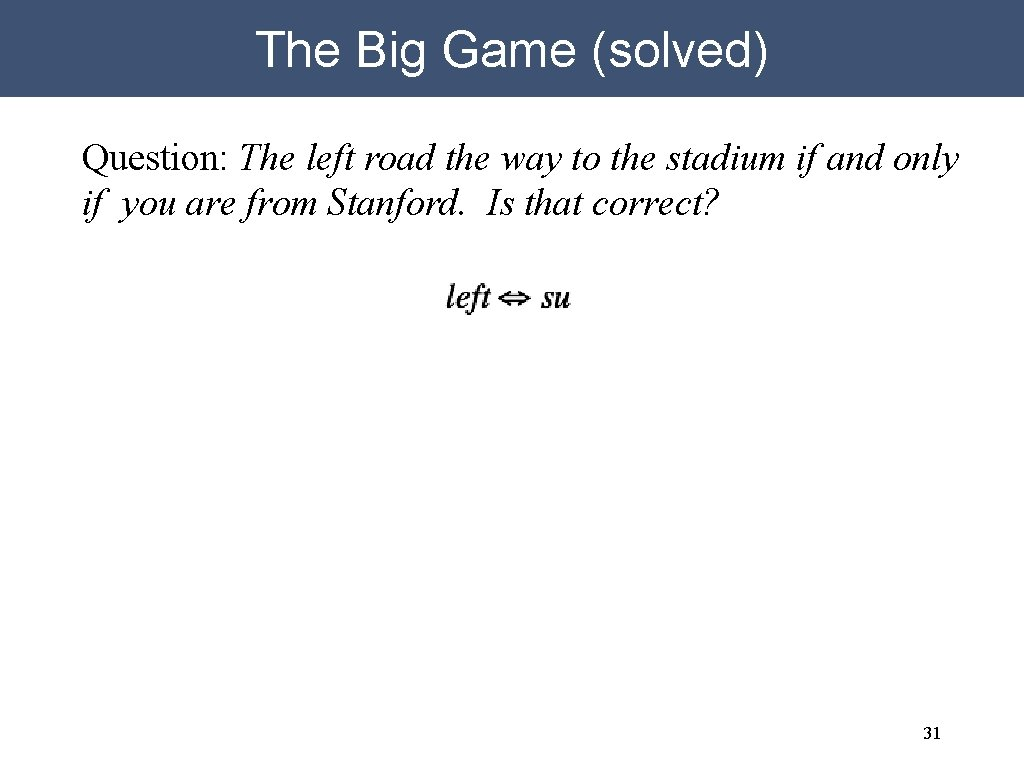The Big Game (solved) Question: The left road the way to the stadium if and only if you are from Stanford. Is that correct? 31